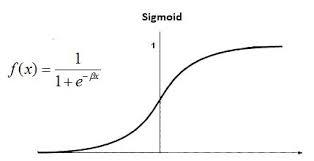Open in App
Not now

• Difficulty Level : Basic
• Last Updated : 10 Jan, 2023

Logistic regression is a classification algorithm used to find the probability of event success and event failure. It is used when the dependent variable is binary(0/1, True/False, Yes/No) in nature. It supports categorizing data into discrete classes by studying the relationship from a given set of labelled data. It learns a linear relationship from the given dataset and then introduces a non-linearity in the form of the Sigmoid function.Logistic regression is also known as Binomial logistics regression. It is based on sigmoid function where output is probability and input can be from -infinity to +infinity. Let’s discuss some advantages and disadvantages of Linear Regression.

My Personal Notes arrow_drop_up